# Ideal number

(diff) ← Older revision | Latest revision (diff) | Newer revision → (diff)

An element of the semi-groupof divisors (cf. Divisor) of the ringof integers of an algebraic number field. The semi-groupis a free commutative semi-group with identity; its free generators are called prime ideal numbers. In modern terminology, ideal numbers are known as integral divisors of. They can be identified in a natural way with the ideals (cf. Ideal) of.

Ideal numbers were introduced in connection with the absence of uniqueness of factorization into prime factors in the ring of integers of an algebraic number field. For every, the factorization of the corresponding divisor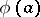into the product of prime ideal numbers can be looked at as a substitute for unique factorization into prime factors if factorization inis not unique.

For example, the ringof integers of the field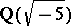consists of the numbers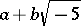with integersand. In this ring, the number 6 has two different factorizations: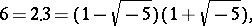where the numbers 2, 3,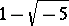, and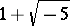are pairwise non-associated irreducible (prime) elements of; thus factorization into irreducible factors inis not unique. However, inthe elements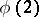,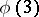,, and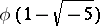are not irreducible; in fact,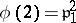,,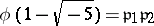,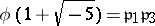, where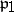,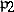and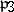are prime ideal numbers in. Thus, the two factorizations of 6 into irreducible factors ingive rise to one and the same factorization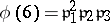in.

The concept of an ideal number was introduced by E. Kummer in connection with his investigation of the arithmetic of cyclotomic fields (see , ). Let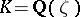be the-th cyclotomic field for some prime numberand letbe the ring of integers of. The ideal numbers forwere defined to be the products of prime ideal numbers, and the latter as the "ideal" prime divisors of natural prime numbers. To construct all the prime ideal numbers contained in a given natural prime number, Kummer's theorem (cf. Kummer theorem) was used. Kummer used the fact thathas basis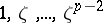over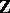to investigate the factorization of the-th cyclotomic polynomial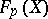in the ring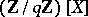. The ideal numbers dividingare in one-to-one correspondence with the irreducible factors ofin(the case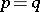required a somewhat different approach). A special method was applied to determine the exponent with which a given prime ideal number occurs in a given. He developed a similar method for creating a theory of divisibility in fields of the form, where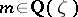.

The extension of the theory of ideal numbers to the case of arbitrary algebraic fields is due mainly to L. Kronecker and R. Dedekind. A division of the theory of ideal numbers into the theory of divisors (the approach of Kronecker) and the theory of ideals begins to appear in their papers. Dedekind associated with every ideal number a unique ideal of the ring, which was defined by him as the subset ofconsisting of 0 together with allthat are divisible by this ideal number. If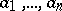are generators for the ideal, then the ideal number corresponding tois the greatest common divisor of the ideal numbers.

Later, the concept of an ideal was extended to the case of an arbitrary ring; rings for which the concepts of an ideal and a divisor coincide are now called Dedekind rings (cf. Dedekind ring).

How to Cite This Entry:
Ideal number. Encyclopedia of Mathematics. URL: http://encyclopediaofmath.org/index.php?title=Ideal_number&oldid=15818
This article was adapted from an original article by L.V. Kuz'min (originator), which appeared in Encyclopedia of Mathematics - ISBN 1402006098. See original article